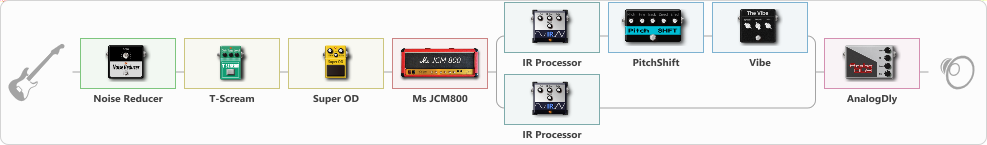# British Heavy

Discussion in 'ToneLib-GFX presets' started by Tony the Metalhead, Dec 6, 2021.

1. ### Tony the MetalheadActive Member

British Heavy

Preset name: British Heavy

Effects chain:Effect: "Noise Reducer" (Dynamics / Filter), active - "yes"
{
"Sens" = 65
"Mode" = Hard
}

Effect: "T-Scream" (Overdrive / Distortion), active - "yes"
{
"Drive" = 5
"Tone" = 60
"Level" = 40
}

Effect: "Super OD" (Overdrive / Distortion), active - "yes"
{
"Drive" = 40
"Tone" = 40
"Level" = 40
}

Effect: "Ms JCM800" (Amp simulators), active - "yes"
{
"Gain" = 100
"Bass" = 20
"Middle" = 25
"Treble" = 100
"Presence" = 80
"Master" = 65
"Level (dB)" = -6
}

Effect: "Splitter" (Dynamics / Filter)
{
"A-Bypass" = Off
"A-Pan" = 0
"A-Level" = 55
"B-Bypass" = Off
"B-Pan" = 0
"B-Level" = 55

'A' branch:
{

Effect: "IR Processor" (Cabinets), active - "yes"
{
"IR" = OD-R112-V30-E906-P10-20
"Low Cut (Hz)" = 0
"Hi Cut (kHz)" = 20.0
"Mix" = 100
"Level (dB)" = 0
}

Effect: "PitchShift" (Modulation / Sfx), active - "yes"
{
"Pitch" = 5
"Fine" = 5
"Tracking" = 25
"Direct" = 100
"Effect" = 25
}

Effect: "Vibe" (Modulation / Sfx), active - "yes"
{
"Speed" = 5.0
"Depth" = 25
"Mix" = 5
}
}
'B' branch:
{

Effect: "IR Processor" (Cabinets), active - "yes"
{
"IR" = OD-R112-V30-E906-P10-20
"Low Cut (Hz)" = 0
"Hi Cut (kHz)" = 20.0
"Mix" = 100
"Level (dB)" = 0
}
}
}

Effect: "AnalogDly" (Delay), active - "yes"
{
"Time" = 333
"Feedback" = 25
"Tone" = 50
"Mix" = 35
}

Note: You will need to download and install the ToneLib-GFX software to use the preset.

File size:
8.5 KB
Views:
2,263